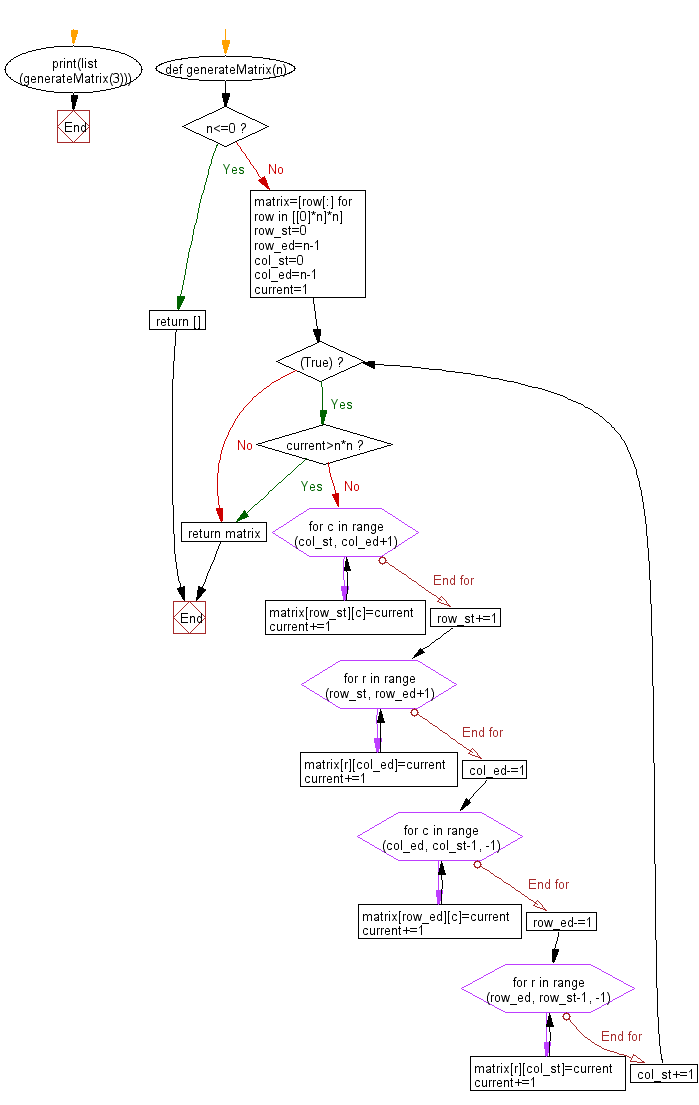﻿ Python Math: Generate a square matrix filled with elements from 1 to n2 in spiral order - w3resource# Python Math: Generate a square matrix filled with elements from 1 to n raised to the power of 2 in spiral order

## Python Math: Exercise-73 with Solution

Write a Python program to generate (given an integer n) a square matrix filled with elements from 1 to n raised to the power of 2 in spiral order.

Sample Solution:-

Python Code:

``````#https://gist.github.com/Ray1988/6806c1b85af70388a277
def generateMatrix(n):
if n<=0:
return []

matrix=[row[:] for row in [*n]*n]

row_st=0
row_ed=n-1

col_st=0
col_ed=n-1
current=1

while (True):
if current>n*n:
break
for c in range (col_st, col_ed+1):
matrix[row_st][c]=current
current+=1
row_st+=1
for r in range (row_st, row_ed+1):
matrix[r][col_ed]=current
current+=1
col_ed-=1
for c in range (col_ed, col_st-1, -1):
matrix[row_ed][c]=current
current+=1
row_ed-=1
for r in range (row_ed, row_st-1, -1):
matrix[r][col_st]=current
current+=1
col_st+=1
return matrix

print(list(generateMatrix(3)))
```
```

Sample Output:

```[[1, 2, 3], [8, 9, 4], [7, 6, 5]]
```

Pictorial Presentation:Flowchart:## Visualize Python code execution:

The following tool visualize what the computer is doing step-by-step as it executes the said program:

Python Code Editor:

Have another way to solve this solution? Contribute your code (and comments) through Disqus.

What is the difficulty level of this exercise?

Test your Programming skills with w3resource's quiz.

﻿

```>>> students = [{'name': 'John', 'score': 98}, {'name': 'Mike', 'score': 94}, {'name': 'Jennifer', 'score': 99}]